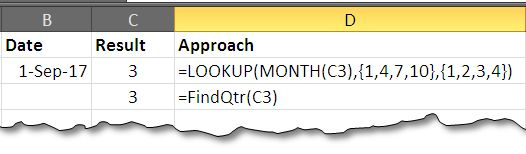# How To Find The Quarter Of YearAccounts finance and marketing people are often concerned with the term “Quarter” in context of their budgeting and marketing assignments. The literal meaning of the word is one-fourth of something and these people use them to donate quarters of a year.

In today’s post we will try to find method that can give us quarters based on a date of the year. We will try out both the formula and the VBA approach to address the problem.

 Month Jan Feb Mar Apr May Jun Jul Aug Sep Oct Nov Dec Quarter 1 2 3 4

The usual setup for finding quarter is that we bunch the months. Finding quarter means we want to find in which bracket does the month fall. So we will create bins and try to match the month with bins to get the quarters.

The formula approach:

Let’s assume we have a date: 02/05/2017 (05th Feb 2017)

Approach No. 01 – Using Lookup Formula:

Using Excel’s function LOOKUP() we can get the following solution:

=LOOKUP(MONTH(B3),{1,4,7,10},{1,2,3,4})

In this formula the bin in created by using an array within the formula: {1,4,7,10} and the next array {1,2,3,4} returns the corresponding quarter number.

MONTH() is used to find the month from the date. The formula works by taking up the month value and matching it with the bins, after match it will return the largest value smaller then the month – in our case it is 1 and the corresponding element is 1 from the second row.

You can make it more fancy by appending the word “Qtr” (using concatenation “&”) or any other word you like.

Approach No. 02 – Using VBA Macro:

The following lines of code when used will return the quarter of the year.

Function FindQtr(dt As Date)

mon = Month(dt)

If mon >= 1 And mon <= 3 Then

FindQtr = 1

ElseIf mon >= 5 And mon <= 6 Then

FindQtr = 2

ElseIf mon >= 7 And mon <= 9 Then

FindQtr = 3

ElseIf mon >= 10 And mon <= 12 Then

FindQtr = 4

End If

End Function

This code returns the same result as that from formula. The code starts by declaring variable Dt as Date. The code uses IF statement to check which quarter does the month belong to and then return corresponding values 14.

Where to put this code:

Press Alt+F11 to open the VBA editor. Then go to Insert > Module that will add module to your file. Now double click Module to open it and paste the code there. Save the file as macro enables sheet and use it. if you want to use it with all the files,  paste it in to your personal personal.xlb and then it will be accessible with all the files.

The result:Companion File:

You can find the companion file from here that contains both types of solutions.